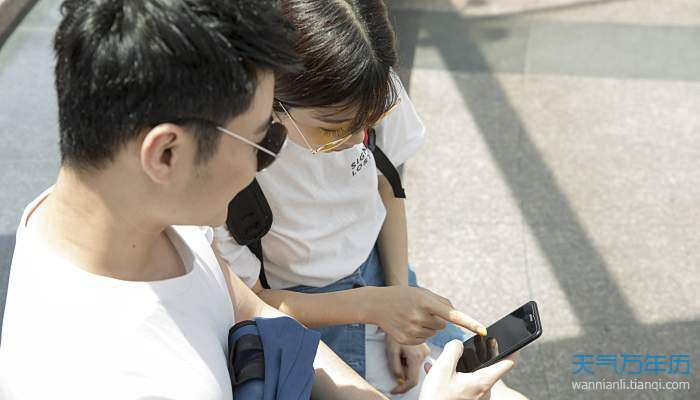# 昵称情侣简短好听 简单好听的情侣网名

2022-01-25  来源：天气万年历  【字体：1.旧街里的酒==老巷里的猫

2.绿衣裙玫瑰巷==白衬衫老胡同

3.暖于心-==动于情

4.深言不离==久言不弃

5.做错要承认==挨打要立正

6.风==等风来

7.深爱不弃==久爱不腻

8.人间不值得==但你最值得

9.晚来天欲雪==能饮一杯无

10.温柔==败给温柔

11.温柔==扑向温柔

12.桃花==满眼尽是桃花

13.斟酌烈酒==唇吻清茶

14.离人散==清歌挽

15.很酷又爱笑==温柔又骄傲

16.两岁就会娇了==三岁就会浪了

17.划船不用桨==一生全靠浪

18.乍见之欢==久处不厌

19.始于心动°==止于枯骨°

20.画一方禁地==囚你于无期

21.看一场不痛不痒的电影==谈一场没有目的的爱情

22.微微一笑很倾城==奈何桥边笑奈何

23.一生如影相随==一世相濡以沫

24.深情不及久伴==厚爱无需多言

25.玲珑骰子安红豆==入骨相思知不知

26.星河漫漫♥==月牙弯弯♥

27.我以后再也不理你了==你上次也是这么说的

28.九月与凉人==十月与安生

29.光阴许我陪你白头==时光许我陪你偕老

30.清九==渡十

31.城南花已落==城北树已枯

32.你若不离==我便不弃

33.治愈系少年♥==病态系少女♥

34.椿兮如梦==湫兮如风

35.南街听风==北街看雨

36.幼儿园大佬==养老院恶魔

37.余生有你♥==请多指教♥

38.七巷公子==九街姑娘

39.故事与他==孤独与酒

40.快说我爱你i==就不说这句i

41.今天要嫁给你==明天要娶回你

42.蓝色的想念==粉红的诱惑

43.他是我的男人☼==她是我的女人☼

44.大手套☂==小手套☂

45.橘子味儿的猫==草莓味儿的狗

46.稚于最初==安于情长

47.七年凉城空浮生==三年空城已离殇

48.生物毁了我的清白==数学毁了我的未来

49.沐北清歌寒==沐南伊人舞

50.野的像风°==傲的像光°51.拥之则安==伴之则暖

52.心不伤不坚强==人不伤不成长

53.左哼哼==右哼哼

54.艺闪艺闪亮晶晶==满天都是张艺兴

55.少年多酷不适合抽烟==少女多美不适合化妆

56.じòぴé帅咾伀ゼ==じòぴé乖咾嘙ゼ

57.你咋还不上天==上天不如上你

58.我负责挣钱养家==我负责貌美如花

59.老子背着书包去打架==老娘穿着校服逛酒吧

60.半世情愿つ==一生守候つ

61.初见°==初心°

62.沙哑情话==匿名情书

63.老婆你好萌==老公你好酷

64.今生未完==来生待续

65.你是年少的欢喜==喜欢的少年是你

66.旧巷少年==西街姑娘

67.余生我还做你妻==余生我还做你君

68.初于青丝==终于白发

69.爱上妳刻骨的温柔==恋上妳铭心的柔弱

70.此女名草有主☼==此男名花有主☼

71.青衫忆笙==素衣白裙

72.睡对了就结婚==睡错了就青春

73.星期八订婚==十三月结婚

74.晚月々==清秋々

75.可爱品格==绅士习性

76.你说黑夜太难熬==我陪你日夜颠倒

77.爱世间万物==万物皆是我

78.银河==心系银河

79.风==挽风

80.长街拥狗==短巷抚猫

81.月色==月色多温柔

82.四月森林==五月天空

83.仅有的温柔==仅存的霸气

84.旺仔甜妹妹==果味怪叔叔

85.怀中猫==养猫人

86.始于脸红==终于眼红

87.书不尽清雨ღ==画不尽晚风ღ

88.京城一匹鹿==首尔小白眼

89.许莫离==许莫弃

90.尘世美==我独醉

91.蓝色的青春==粉色的回忆

92.阿狸㏒==桃子㏕

93.紫色羁绊==蓝色妖姬

94.鸢飞戾天==鱼跃于渊

95.初心不改==真心不变

96.い时光｀流年°==い时光｀爱恋°

97.地久天长==白头偕老

98.空失心==旧爱人

99.荣幸==我的荣幸

100.清酒暖心==烈酒醉人